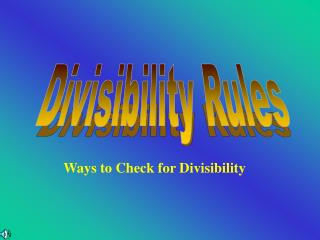DownloadDownload PresentationWays to Check for Divisibility

# Ways to Check for Divisibility

Download Presentation## Ways to Check for Divisibility

- - - - - - - - - - - - - - - - - - - - - - - - - - - E N D - - - - - - - - - - - - - - - - - - - - - - - - - - -
##### Presentation Transcript

1. Divisibility Rules Ways to Check for Divisibility

2. Objective • I will learn divisibility rules in order to help me find factors of whole numbers.

3. Dividing By 1 • All numbers are divisible by 1

4. Now You Try • 578 • 398 • 48 • 1903 • 490 Are these numbers divisible by 1?

5. Dividing by 2 • All even numbers are divisible by 2 • Even numbers are numbers that end with either 2, 4, 6, 8, or 0

6. Now You Try • 458 • 1279 • 759 • 555 • 1050 Are these numbers divisible by 2?

7. Dividing by 3 • Add up the digits of the number • If that number is divisible by 3, then the original number is • If your sum is still a big number, continue to add the digits

8. 7 3 8 • For example, take the number 7 + 3 + 8 = Dividing by 3 • Add up the digits of the number 18 • If that number is divisible by 3, then the original number is Is 18 divisible by 3? • If your sum is still a big number, continue to add the digits 1 + 8 = 9 Is 9 divisible by 3?

9. Now You Try • 639 • 56 • 86 • 360 • 468 Are these numbers divisible by 3?

10. Dividing by 4 • If the last 2 digits together are divisible by 4

11. Now You Try • 584 • 261 • 56 • 920 • 767 Are these numbers divisible by 4?

12. Dividing by 5 • If the number ends in 5 or 0

13. Now You Try • 554 • 6890 • 345 • 902 • 845 Are these numbers divisible by 5?

14. Dividing by 6 • If the number is divisible by 2, and . . . • If the number is divisible by 3

15. Now You Try • 897 • 258 • 630 • 345 • 84 Are these numbers divisible by 6?

16. Dividing by 7

17. Now You Try • 578 • 398 • 48 • 1903 • 490 Are these numbers divisible by 7?

18. So what type of number does it have to be? Dividing by 8 • If the last three digits are divisible by 8 • If the number is divisible by 2, • then by 2 again, and then by 2 again

19. Now You Try • 568 • 396 • 48 • 1903 • 490 Are these numbers divisible by 8?

20. Dividing by 9 • Similar to dividing by 3 • Add up digits • If that number is divisible by 9 then your number is divisible by 9

21. 6 9 + + 5 2 + + 4 1 = + Dividing by 9 • For example, take the number 924,561 • Add up the digits of the number 27 • If that number is divisible by 3, then the original number is Is 27 divisible by 9? • If your sum is still a big number, continue to add the digits 2 + 7 = 9 Is 9 divisible by 9?

22. Now You Try • 578 • 398 • 48 • 1903 • 490 Are these numbers divisible by 9?

23. Dividing by 10 • If the number ends with a 0

24. Now You Try • 578 • 398 • 48 • 1903 • 490 Are these numbers divisible by 10?

25. Dividing by 11

26. Now You Try • 578 • 398 • 48 • 1903 • 490 Are these numbers divisible by 11?

27. Divisible by 1 Divisible by 2 Divisible by 3 Divisible by 4 Divisible by 5 Divisible by 6 Divisible by 6 Divisible by 8 Divisible by 9 Divisible by 10 Divisible by 11 REVIEW

28. Assignment Tell what each number is divisible by, either 2, 3, 4, 5, 6, 7, 8, 9, 10, or 11 • 3870 • 7896 • 69095 • 4892 • 3487 • 186 • 85 • 69 • 298 • 747 • 952 • 3650 • 2738 • 1132 • 5084

29. That's all Folks!